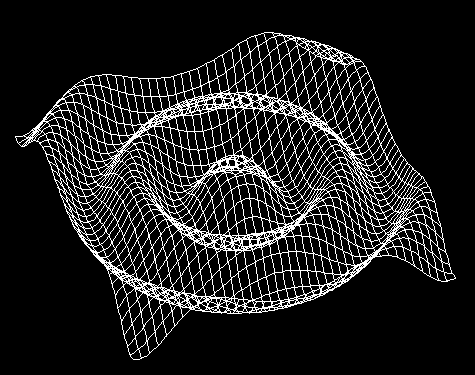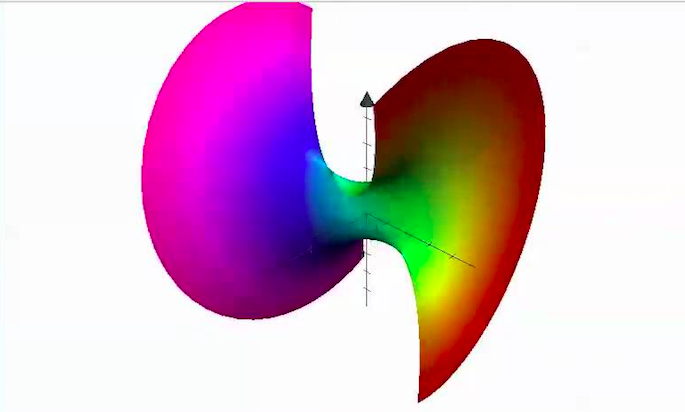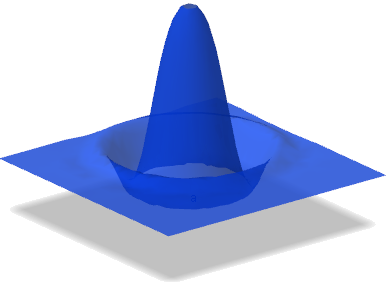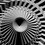# Multi - Dimensional Sine/Cos Waves

Here's an interesting thing I thought up a while ago that I thought would be interesting for all of you. I would like some answers if possible, please!

Take a cos wave - a standard cos wave. Rotate it around the y axis, and you get a 3d cos wave, like ripples in a pond. Now, take a sound wave in action, and graph it as it moves (as it would travel in space), rotated around the y axis. Here's what you get:So cool! Now, theoretically, based on this rotation idea, you can rotate this 3d wave around the Z axis, and get a 4 - dimensional Sin wave. below is a 3 - dimensional projection of a 4 - dimensional sine wave created in grapher:I guess this might be related to the finding of higher dimensional standards - a point is 0d. A moving point makes a line, 1d. Moving line, square, 2d. A moving square, cube, 3d. A moving cube, 4d, hypercube, etc.

I guess you could continue to find even higher dimensional waves... you just probably couldn't graph them easily. :D

Welp, sorry - guess I'm not done. Area of a square is L^2, Volume of a cube is L^3, Volume (I don't know what else to call it - what is 4D volume called) is L^4. Area of circle is πR^2, Volume of a sphere is 4/3πR^3, and Volume (again, Idk what to call it) of a so called "hypersphere" is π^2r^4 all over 2. Now, using the fundamental theorem of calculus, we can find the area under a cos wave from the points 0 to 2π (one phase) with respect to x:Using the power rule and evaluating from 0 to 2π, we get 4π as the area under this wave from 0 to 2π. (Not too good with LaTeX, so I didn't show all of my steps) Now, my question is how to find the area (or Volume, rather) underneath the wave rotated around the y axis, not as it fluctuates, but at a single point in time - the volume under the ripples to the XZ plane.This would definitely involve multivariable calculus and a ton of time... this would be so complex. thinking in 2d, we find the area under a curve by thinking of an infinite amount of rectangles that we fit under it - for a 3d wave, we'd have to find an infinite amount of rectangular prisms to fit underneath... would this qualify as multivariable calculus? I honestly don't have a clue :D

Then, what about the area under a 4d sine wave? we'd be dealing with an infinite number of hyper - prisms fit under a hyper curve...

My head hurts. I honestly don't know how to approach this. Is something like this possible in even higher dimensions as well? I mean, we can do it in 2D, it is theoretically possible in 3D, but in things like 4D, is it even feasible to try? And of what use would it be if it was possible?

Any suggestions or ideas would be greatly appreciated - this is a pretty difficult problem!Note by Hunter Edwards
3 years, 7 months ago

This discussion board is a place to discuss our Daily Challenges and the math and science related to those challenges. Explanations are more than just a solution — they should explain the steps and thinking strategies that you used to obtain the solution. Comments should further the discussion of math and science.

When posting on Brilliant:

• Use the emojis to react to an explanation, whether you're congratulating a job well done , or just really confused .
• Ask specific questions about the challenge or the steps in somebody's explanation. Well-posed questions can add a lot to the discussion, but posting "I don't understand!" doesn't help anyone.
• Try to contribute something new to the discussion, whether it is an extension, generalization or other idea related to the challenge.
• Stay on topic — we're all here to learn more about math and science, not to hear about your favorite get-rich-quick scheme or current world events.

MarkdownAppears as
*italics* or _italics_ italics
**bold** or __bold__ bold
- bulleted- list
• bulleted
• list
1. numbered2. list
1. numbered
2. list
Note: you must add a full line of space before and after lists for them to show up correctly
paragraph 1paragraph 2

paragraph 1

paragraph 2

[example link](https://brilliant.org)example link
> This is a quote
This is a quote
    # I indented these lines
# 4 spaces, and now they show
# up as a code block.

print "hello world"
# I indented these lines
# 4 spaces, and now they show
# up as a code block.

print "hello world"
MathAppears as
Remember to wrap math in $$ ... $$ or $ ... $ to ensure proper formatting.
2 \times 3 $2 \times 3$
2^{34} $2^{34}$
a_{i-1} $a_{i-1}$
\frac{2}{3} $\frac{2}{3}$
\sqrt{2} $\sqrt{2}$
\sum_{i=1}^3 $\sum_{i=1}^3$
\sin \theta $\sin \theta$
\boxed{123} $\boxed{123}$

## Comments

Sort by:

Top Newest

Isn't the area under a cos wave just 2? I don't see where you're getting the equation you wrote down. I would set up the integral like this: 2*$\int_{0}^{\pi} cos(x)$

(You have to multiply by 2 because the area is negative after x=pi)

You seem to just be finding the area of cos + 2 from 0 to 2pi, which is the same as the area under a line from 0 to 2pi (since the average value of the cosine is 0 from 0 to 2pi)

- 3 years, 7 months ago

Log in to reply

Right. Sorry for the mistake - my stuff has been mixed up lately >:( What you said makes sense, as the cos function simply loops repeatedly, and the upper parts account for the lower parts. At π, y would equal -1. I want to evaluate for an entire cycle, and I added two to remove the parts where Y is negative, thus giving a true area under the curve... In addition to this, there would be no negative sections on the 3-D graphing.

Any ideas for anything in the further - down section of my note?

- 3 years, 7 months ago

Log in to reply

Well, with regards to 3D, we can use polar coordinates to find the area under the curve pretty easily. You would set up the integral like this $\int _{ 0}^{2\pi}{\int _{0}^{ \pi}{cos( \theta )rdr}\theta}$

The r factor is just because you're integrating with polar coordinates, the cosine is the height of the curve, and the outer integral is to account for the rotation around the origin. The inner integral is more arbitrary - it just gets the volume of the first wave. You could get the second wave or third wave or whatever by changing the bounds and maybe switching the signs. The higher dimensions are a bit more challenging, though!

I guess one thing to consider with the 4D curves is exactly how you're defining them. I think there are probably a couple of ways to interpret '4-D cos wave'. Once you have a solid mathmatical definition, it should be easier to solve the problem. For 3D, you have a very good definition, rotating it around the origin. (Edit: another possible definition could be y=cos(x), with the z-dimension always being a constant for a given y and x). But with 4D you're being very vague, which is understandable, because, well, it's 4D. I think the best next step to solving the problem is to better formulate it (though, don't get me wrong, your post is excellent).

- 3 years, 7 months ago

Log in to reply

Thanks so much! I was pretty discouraged after trying for a while. I'll have to work on defining a 4D curve - I'll let you know if I get any results :)

- 3 years, 7 months ago

Log in to reply

×

Problem Loading...

Note Loading...

Set Loading...